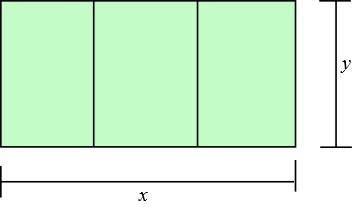# Farmer Brown has 300 yards of fencing with which to build a rectangular corral. He wants to...

## Question:

Farmer Brown has 300 yards of fencing with which to build a rectangular corral. He wants to divide it evenly into three pens, so he adds in two divider fences, as shown below. answer the following:

a) Write the area of the corral as a function of {eq}x{/eq}, so {eq}A(x)={/eq}?

b) Determine the maximum area (to the nearest whole yard) enclosed by the corral. So the maximum area equals how many square yards?## Maximizing Area of a Rectangle:

A rectangle is a 2-dimensional figure which has 4 sides. The pair of opposite sides of a rectangle are parallel to each other and are of the same length. The area of a rectangle with length {eq}l{/eq} and width {eq}w{/eq} is {eq}l{\times}w {/eq}.

For a function of one variable, {eq}f(x){/eq}, the maxima can be found by differentiating {eq}f(x){/eq} with respect to {eq}x{/eq} and then equating it to 0. The value(s) of {eq}x{/eq} which satisfies this equation gives us the points at which {eq}f(x){/eq} is a maximum.

Let the width of the corral be {eq}x{/eq} yards.

Let the length the corral be {eq}y{/eq} yards.

As each pen shares a common wall, the length of all fencing is

{eq}2x+4y=300 \\ y=\frac{300-2x}{4} \\ y=\frac{150-x}{2} \\ {/eq}

a) The area of the corral will be

{eq}A(x)=l{\times}w \\ A(x)=x{\times}y \\ A(x)=x{\times}\frac{150-x}{2} \\ A(x)=\frac{150x-x^2}{2} {/eq}

b) To get the maximum area we will differentiate A with respect to {eq}x{/eq} and equate it to zero. This critical point will show the value of {eq}x{/eq} which gives the maximum area.

{eq}\begin{align} \frac{dA}{dx}&=\frac{d(\frac{150x-x^2}{2})}{dx} \\ \frac{dA}{dx}&=\frac{150-2x}{2} \\ 0&=\frac{150-2x}{2} \\ x&=\frac{150}{2} \\ x&=75 \end{align} {/eq}

The maximum area of the corral is

{eq}A(75)=\frac{150(75)-(75)^2}{2}\\ A=2812.5 {/eq}

Hence, the maximum area (to the nearest whole square yard) enclosed by the corral is 2813 square yards.